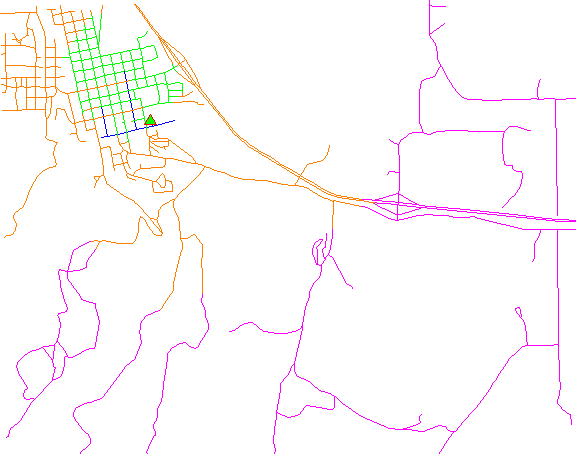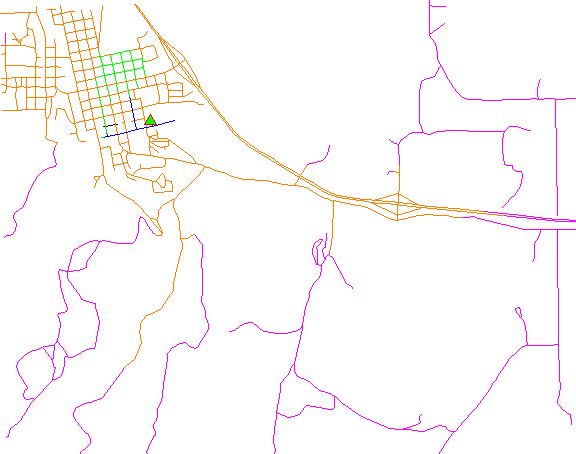## NAME

v.net.iso - Splits subnets for nearest centers by cost isolines.
Splits net to bands between cost isolines (direction from center). Center node must be opened (costs >= 0). Costs of center node are used in calculation.

## KEYWORDS

vector, network, cost allocation, isolines

## SYNOPSIS

v.net.iso
v.net.iso --help
v.net.iso [-tgu] input=name output=name [method=string] center_cats=range costs=integer[,integer,...] arc_layer=string arc_type=string[,string,...] node_layer=string [arc_column=name] [arc_backward_column=name] [node_column=name] [turn_layer=string] [turn_cat_layer=string] [--overwrite] [--help] [--verbose] [--quiet] [--ui]

### Flags:

-t
Use turntable
-g
Use geodesic calculation for longitude-latitude locations
-u
Create unique categories and attribute table
Default: one category for each iso-band
--overwrite
Allow output files to overwrite existing files
--help
Print usage summary
--verbose
Verbose module output
--quiet
Quiet module output
--ui
Force launching GUI dialog

### Parameters:

input=name [required]
Name of input vector map
Or data source for direct OGR access
output=name [required]
Name for output vector map
method=string
Use costs from centers or costs to centers
Options: from, to
Default: from
center_cats=range [required]
Category values
Categories of centers (points on nodes) to which net will be allocated, layer for this categories is given by nlayer option
costs=integer[,integer,...] [required]
Costs for isolines
arc_layer=string [required]
Arc layer
Vector features can have category values in different layers. This number determines which layer to use. When used with direct OGR access this is the layer name.
Default: 1
arc_type=string[,string,...] [required]
Arc type
Input feature type
Options: line, boundary
Default: line,boundary
node_layer=string [required]
Node layer
Vector features can have category values in different layers. This number determines which layer to use. When used with direct OGR access this is the layer name.
Default: 2
arc_column=name
Arc forward/both direction(s) cost column (number)
arc_backward_column=name
Arc backward direction cost column (number)
node_column=name
Node cost column (number)
turn_layer=string
Layer with turntable
Relevant only with -t flag
Default: 3
turn_cat_layer=string
Layer with unique categories used in turntable
Relevant only with -t flag
Default: 4

## DESCRIPTION

v.net.iso splits a network into bands between cost isolines (distance from center). Center nodes must be opened (costs >= 0). The costs of center nodes are used in the calculation.

Costs may be either line lengths, or attributes saved in a database table. These attribute values are taken as costs of whole segments, not as costs to traverse a length unit (e.g. meter) of the segment. For example, if the speed limit is 100 km / h, the cost to traverse a 10 km long road segment must be calculated as
length / speed = 10 km / (100 km/h) = 0.1 h.
Supported are cost assignments for both arcs and nodes, and also different costs for both directions of a vector line. For areas, costs will be calculated along boundary lines.

The input vector needs to be prepared with v.net operation=connect in order to connect points representing center nodes to the network.

The nearest center can be determined using either costs from the nearest center or costs to the nearest center with option method.

By default, the iso band number is used as category value for output lines. With the -u flag, output lines become unique categories and an attribute table is created with the fields cat, ocat, center, isonr, isolbl. The ocat field holds the original line category in arc_layer, the center field holds the center category in node_layer, the isonr field holds the iso band number and the isolbl field holds a label for the isoband. Additionally, original line categories are copied from the input arc_layer to layer 2 in the output, together with any attribute table.

Application of flag -t enables a turntable support. This flag requires additional parameters turn_layer and turn_cat_layer that are otherwise ignored. The turntable allows to model e.g. traffic code, where some turns may be prohibited. This means that the input layer is expanded by turntable with costs of every possible turn on any possible node (intersection) in both directions. Turntable can be created by the v.net module. For more information about turns in the vector network analyses see wiki page.

## NOTES

Nodes and arcs can be closed using cost = -1.

Nodes must be on the isolines.

## EXAMPLES

The map must contain at least one center (point) on the vector network which can be patched into with v.net.

Isonetwork using distance:Isonetwork using time:#### Subdivision of a network using distance:

```# Spearfish

# start node:
echo "591280.5|4926396.0|1" | v.in.ascii in=- out=startnode
g.copy vect=roads,myroads

# connect point to network
v.net myroads points=startnode out=myroads_net op=connect thresh=200

# define iso networks using distance:
v.net.iso input=myroads_net output=myroads_net_iso center_cats=1-100000 costs=1000,2000,5000
```
The network is divided into 4 categories:
```v.category myroads_net_iso option=report
# ... reports 4 categories:
#cat | distance from point in meters
#1          0 - < 1000
#2       1000 - < 2000
#3       2000 - < 5000
#4             >= 5000
```
To display the result, run for example:
```g.region n=4928200 s=4922300 w=589200 e=596500
d.mon x0
d.vect myroads_net_iso col=blue   cats=1
d.vect myroads_net_iso col=green  cats=2
d.vect myroads_net_iso col=orange cats=3
d.vect myroads_net_iso col=magenta  cats=4
d.vect myroads_net col=red icon=basic/triangle fcol=green size=12 layer=2
```

#### Subdivision of a network using traveling time:

Prepare the network as above:
```# Spearfish

# start node:
echo "591280.5|4926396.0|1" | v.in.ascii in=- out=startnode
g.copy vect=roads,myroads

# connect point to network
v.net myroads points=startnode out=myroads_net op=connect thresh=200
```
Define costs as traveling time dependent on speed limits:
```# set up costs

# create unique categories for each road in layer 3
v.category in=myroads_net out=myroads_net_time opt=add cat=1 layer=3 type=line

# add new table for layer 3
v.db.addtable myroads_net_time layer=3 col="cat integer,label varchar(43),length double precision,speed double precision,cost double precision,bcost double precision"

# copy road type to layer 3
v.to.db myroads_net_time layer=3 qlayer=1 opt=query qcolumn=label columns=label

# upload road length in miles
v.to.db myroads_net_time layer=3 type=line option=length col=length unit=miles

# set speed limits in miles / hour
v.db.update myroads_net_time layer=3 col=speed val="5.0"
v.db.update myroads_net_time layer=3 col=speed val="75.0" where="label='interstate'"
v.db.update myroads_net_time layer=3 col=speed val="75.0" where="label='primary highway, hard surface'"
v.db.update myroads_net_time layer=3 col=speed val="50.0" where="label='secondary highway, hard surface'"
v.db.update myroads_net_time layer=3 col=speed val="25.0" where="label='light-duty road, improved surface'"
v.db.update myroads_net_time layer=3 col=speed val="5.0" where="label='unimproved road'"

# define traveling costs as traveling time in minutes:

# set forward costs
v.db.update myroads_net_time layer=3 col=cost val="length / speed * 60"
# set backward costs
v.db.update myroads_net_time layer=3 col=bcost val="length / speed * 60"

# define iso networks using traveling time:
v.net.iso input=myroads_net_time output=myroads_net_iso_time arc_layer=3 node_layer=2 arc_column=cost arc_backward_column=bcost center_cats=1-100000 costs=1,2,5
```
To display the result, run for example:
```# add table with labels and coloring
v.db.addtable myroads_net_iso_time columns="cat integer,trav_time varchar(20),GRASSRGB varchar(11)"
# labels
v.db.update map=myroads_net_iso_time layer=1 column=trav_time value="0 - 1" where="cat = 1"
v.db.update map=myroads_net_iso_time layer=1 column=trav_time value="1 - 2" where="cat = 2"
v.db.update map=myroads_net_iso_time layer=1 column=trav_time value="2 - 5" where="cat = 3"
v.db.update map=myroads_net_iso_time layer=1 column=trav_time value="> 5" where="cat = 4"
# colors
# cats=1: blue
v.db.update map=myroads_net_iso_time layer=1 column=GRASSRGB value="000:000:255" where="cat = 1"
# cats=2: green
v.db.update map=myroads_net_iso_time layer=1 column=GRASSRGB value="000:255:000" where="cat = 2"
# cats=3: orange
v.db.update map=myroads_net_iso_time layer=1 column=GRASSRGB value="255:128:000" where="cat = 3"
# cats=4: magenta
v.db.update map=myroads_net_iso_time layer=1 column=GRASSRGB value="255:000:255" where="cat = 4"

# show results
g.region n=4928200 s=4922300 w=589200 e=596500
d.mon x0
d.vect myroads_net_iso_time layer=1 -a rgb_col=GRASSRGB
d.vect myroads_net col=red icon=basic/triangle fcol=green size=12 layer=2
```

## SEE ALSO

d.path, v.net, v.net.alloc, v.net.path, v.net.salesman, v.net.steiner, v.patch

## AUTHOR

Radim Blazek, ITC-Irst, Trento, Italy
Documentation: Markus Neteler, Markus Metz

### TURNS SUPPORT

The turns support was implemnented as part of GRASS GIS turns cost project at Czech Technical University in Prague, Czech Republic. Eliska Kyzlikova, Stepan Turek, Lukas Bocan and Viera Bejdova participated at the project. Implementation: Stepan Turek Documentation: Lukas Bocan Mentor: Martin Landa

Last changed: \$Date\$

## SOURCE CODE

Available at: v.net.iso source code (history)

© 2003-2019 GRASS Development Team, GRASS GIS 7.6.2dev Reference Manual